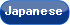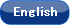Please use this identifier to cite or link to this item: `http://hdl.handle.net/10119/14768`

 Title: Unfolding and Dissection of Multiple Cubes Authors: Abel, ZachBallinger, BranDemaine, Erik DDemaine, Martin LErickson, JeffHesterberg, AdamIto, HiroKostitsyana, IrinaLynch, JaysonUehara, Ryuhei Keywords: polyominorep-tilerep-cubedevelopment of cube Issue Date: 2016 Publisher: 19th Japan Conference on Discrete and Computational Geometry, Graphs, and Games (JCDCGGG 2016) Magazine name: 19th Japan Conference on Discrete and Computational Geometry, Graphs, and Games (JCDCGGG 2016) Abstract: A polyomino is a “simply connected” set of unit squares introduced by Solomon W. Golomb in 1954. Since then, a set of polyominoes has been playing an important role in puzzle society (see, e.g., [3, 1]). In Figure 82 in , it is shown that a set of 12 pentominoes exactly covers a cube that is the square root of 10 units on the side. In 1962, Golomn also proposed an interesting notion called “rep-tiles”: a polygon is a reptile of order k if it can be divided into k replicas congruent to one another and similar to the original (see [2, Chap 19]). These notions lead us to the following natural question: is there any polyomino that can be folded to a cube and divided into k polyominoes such that each of them can be folded to a (smaller) cube for some k? That is, a polyomino is a rep-cube of order k if it is a net of a cube, and it can be divided into k polyominoes such that each of them can be folded to a cube. If each of these k polyominoes has the same size, we call the original polyomino regular rep-cube of order k. In this paper, we give an affirmative answer. We first give some regular rep-cubes of order k for some specific k. Based on this idea, we give a constructive proof for a series of regular rep-cubes of order 36gk'^2 for any positive integer k' and an integer g in {2; 4; 5; 8; 10; 50}. That is, there are infinitely many k that allow regular rep-cubes of order k. We also give some non-regular rep-cubes and its variants. Rights: Zach Abel, Bran Ballinger, Erik D. Demaine, Martin L. Demaine, Jeff Erickson, Adam Hesterberg, Hiro Ito, Irina Kostitsyana, Jayson Lynch, and Ryuhei Uehara, 19th Japan Conference on Discrete and Computational Geometry, Graphs, and Games (JCDCGGG 2016), 2016. URI: http://hdl.handle.net/10119/14768 Material Type: author Appears in Collections: b11-1. 会議発表論文・発表資料 (Conference Papers)

Files in This Item:

File Description SizeFormat